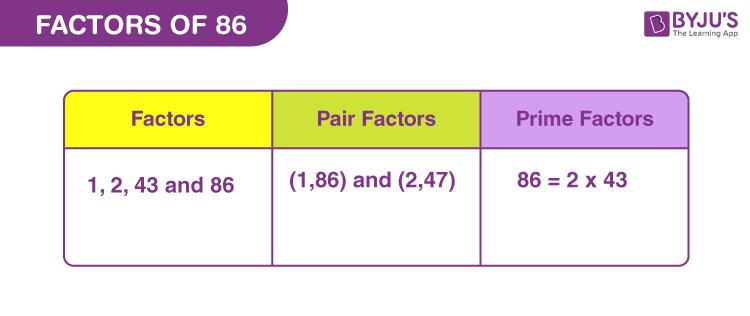# Factors of 86

In Maths, factors of 86 are the real numbers but not fraction. If the product of two whole numbers is equal to 86, then they are the required factors. We can also say, a factor of 86 divides the original number into equal parts. Since 86 is a composite number, it has more than two factors.

Finding the factors is an easy method. We have to perform simple division methods. Hence, we will be able to evaluate the factors, pair factors and prime factors in the following form:Now let us learn how to find these factors.

## How to Find Factors of 86?

The factor of 86 breaks the original number into equal numbers of parts. By factorising, we mean finding the product of two or more numbers that will result in the original number. Now, if the original number has been divided by any of its factors, then the resultant value is a whole number. Let us see how.

86 ÷ 1 = 86

86 ÷ 2 = 43

86 ÷ 43 = 2

86 ÷ 86 = 1

We can see, there is no remainder left after each division and the quotient is a whole number. Thus, the factors of 86 are 1, 2, 43, and 86.

## Pair Factors of 86

When two integers are multiplied and the resulting value is 86, then the pair of integers are called pair factors of 86.

1 × 86 = 86

2 x 43 = 86

Therefore, the pair factors are (1, 86) and (2, 43).

Similarly, we can write the negative pair factors that will result in the original number, after multiplication.

-1 × -86 = 86

-2 × -43 = 86

Therefore, the negative pair factors are (-1, -86) and (-2, -43).

## Prime Factorisation of 86

Prime factorisation of 86 represents the product of prime numbers that results in the original number. Those prime numbers are called prime factors that can divide the original number, uniformly.

Let us list down the prime numbers from 1 to 86, to find the prime factors.

List of prime numbers (Between 1 and 86) :

 2, 3, 5, 7, 11, 13, 17, 19, 23, 29, 31, 37, 41, 43, 47, 53, 59, 61, 67, 71, 73, 79, 83

Step 1: 86 is the even number. Thus it is divisible by 2.

86/2 = 43

Step 2: Now from the above list of prime numbers, we can see, 43 itself is a prime number, hence,

43/43 = 1

1 is not divisible by any prime number. Therefore, the prime factors of 86 are 2 and 43.

 Prime factorisation of 86 = 2 x 43

## Solved Examples

Q.1: Find the sum of all the factors of 86. Also, find the average.

Solution: The factors of 86 are 1, 2, 43, and 86 .

Sum = 1 + 2 + 43 + 86 = 132

Therefore, 132 is the required sum.

Since, sum of all the factors = 132

Number of total factors = 4

Hence,

Average = Sum/Total factors = 132/4 = 33

Q.2: What is the greatest common factor of 42 and 86?

Answer: Let us first write the factors of both the numbers.

42 → 1, 2, 3, 6, 7, 14, 21, 42

86 → 1, 2, 43, 86

Therefore, greatest common factor (GCF) of 42 and 86 = 2

Q.3: What are the common factors of 86, 88 and 85?

85 → 1, 5, 17, 85

86 → 1, 2, 43, 86

88 → 1, 2, 4, 8, 11, 22, 44, 88

Therefore, Common factor is only 1.

## Frequently Asked Questions on Factors of 86

### How many factors does 86 have?

There are a total of four factors of 86. These factors are 1, 2, 43, and 86.

### What are prime factors of 86?

The prime factorisation of 86 is: 2 x 43.

### What are the multiples of 86?

The first ten multiples of 86 are 86, 172, 258, 344, 430, 516, 602, 688, 774 and 860.

### Is 86 a prime number or composite number?

86 is a composite number, since it has more than two factors. Prime numbers have only two factors.

### Is 86 a perfect square?

The square root of the perfect square should be a whole number. Thus, 86 is not a perfect square.